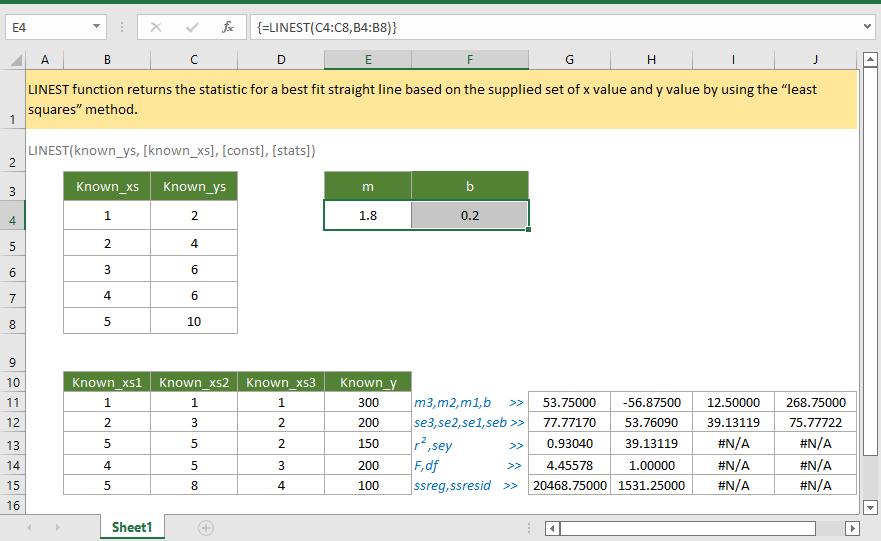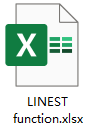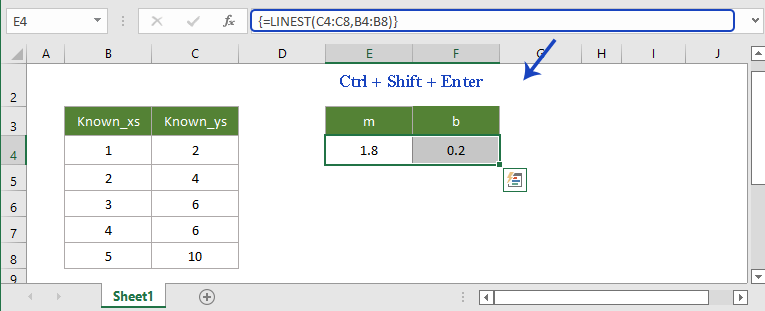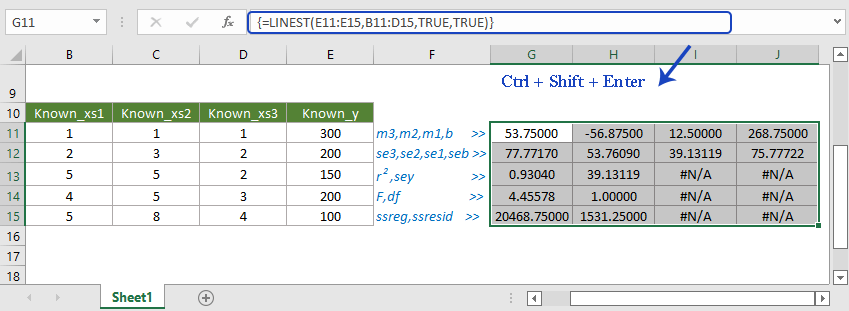## Excel LINEST Function### Description

The Excel LINEST function returns the statistic for a best fit straight line based on the supplied set of x value and y value by using the “least squares” method. The function returns an array of constant values.

The equation for the line is

y = mx + b

where

x is the independent variable;

y is the dependent variable;

m is the slope (gradient) of the line;

b is a constant, equal to the value of y when x = 0

or

y = m1x1+m2x2+…+b

where

the x's are the independent variable ranges;

y is the dependent variable;

the m's are constant multipliers for each x range;

b is a constant.

### Syntax and arguments

Formula syntax

LINEST(known_ys, [known_xs], [const],[stats])

Arguments

• Known_ys: Required, an array of known y values.
• Known_xs: Optional, an array of one or more sets of known x values.
--If provided, the array length of known_xs should be the same asknown_ys.
--If omitted, takes the known_xs array on default value {1,2,3,…}.
• Const: Optional, a logical argument that determines how the constant “b” is treated in the equation y = m1x1+m2x2+…+b
--If it is TRUE, the constant “b” is treated normally.
--If it is FALSE, the constant “b” is 0 (zero), and the equation is adjusted to y = mx.
• Stats: Optional, a logical argument that determines whether to return additional regression statistics.
--If it is FALSE or omitted, the function just returns the array of values {mn,mn-1,…m1,b}.
--If it is TRUE, the function returns additional regression statistics {mn,mn-1,...,m1,b;sen,sen-1,...,se1,seb;r2,sey;F,df;ssreg,ssresid}, which list in the table below:
•  Statistic Description mn,mn-1,…m1 The array of constant multipliers for the straight line equation b The constant value of y when x=0 sen,sen-1,...,se1 The standard error values for the coefficients m1,m2,...,mn seb The standard error value for the constant b (seb = #N/A when const is FALSE) r2 The coefficient of determination sey The standard error for the y estimate f F statistic (F-observed value) df Degrees of freedom ssreg Regression sum of squares ssresid Residual sum of squares

Return Value

The LINEST function returns an array of values.

Remark

The LINEST function returns an array, you must enter it as a multi-cell array formula and press CTRL + Shift + Enter keys to get the results.

Version

Excel 2003 or later

### Usage and Examples

Example 1: for one set of known_xs, and ignore const and stats

In the list B4:B8, contains the known_xs, in the list C4:C8, contains the know_ys, now select cells E4:F4, then type below formula to the formula bar:

=LINEST(C4:C8,B4:B8)

Press Ctrl + Shift + Enter key, the array of results gets out.Example 2: for two or more sets of known_xs and const and stats are TRUEs

In the list B11:D15, contains the known_xs, in the list E11:E15, contains the know_ys, const and stats are TRUEs, select range G11:J15, then type below formula to the formula bar:

=LINEST(E11:E15,B11:D15,TRUE,TRUE)

Press Ctrl + Shift + Enter key, the array of results gets out.#### Relative Functions:

• Excel ROUNDOWN Function
The ROUNDDOWN function rounds a number down (toward zero) to a specified number of digits.

### The Best Office Productivity Tools

#### Kutools for Excel - Helps You To Stand Out From Crowd

 Popular Features: Find, Highlight or Identify Duplicates  |  Delete Blank Rows  |  Combine Columns or Cells without Losing Data  |  Round without Formula ... Super VLookup: Multiple Criteria  |  Multiple Value  |  Across Multi-Sheets  |  Fuzzy Lookup... Adv. Drop-down List: Easy Drop Down List  |  Dependent Drop Down List  |  Multi-select Drop Down List... Column Manager: Add a Specific Number of Columns  |  Move Columns  |  Toggle Visibility Status of Hidden Columns  |  Compare Columns to Select Same & Different Cells ... Featured Features: Grid Focus  |  Design View  |  Big Formula Bar  |  Workbook & Sheet Manager | Resource Library (Auto Text)  |  Date Picker  |  Combine Worksheets  |  Encrypt/Decrypt Cells  |  Send Emails by List  |  Super Filter  |  Special Filter (filter bold/italic/strikethrough...) ... Top 15 Toolsets:  12 Text Tools (Add Text, Remove Characters ...)  |  50+ Chart Types (Gantt Chart ...)  |  40+ Practical Formulas (Calculate age based on birthday ...)  |  19 Insertion Tools (Insert QR Code, Insert Picture from Path ...)  |  12 Conversion Tools (Numbers to Words, Currency Conversion ...)  |  7 Merge & Split Tools (Advanced Combine Rows, Split Excel Cells ...)  |  ... and more

Kutools for Excel Boasts Over 300 Features, Ensuring That What You Need is Just A Click Away...#### Office Tab - Enable Tabbed Reading and Editing in Microsoft Office (include Excel)

• One second to switch between dozens of open documents!
• Reduce hundreds of mouse clicks for you every day, say goodbye to mouse hand.
• Increases your productivity by 50% when viewing and editing multiple documents.
• Brings Efficient Tabs to Office (include Excel), Just Like Chrome, Edge and Firefox.